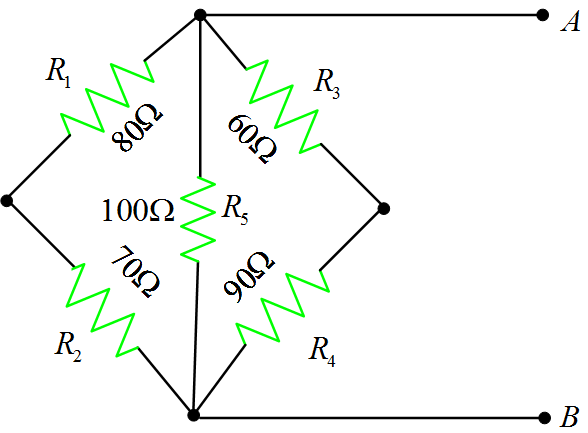Home / Circuits with Matlab / Equivalent Resistance Calculation in a Complex Circuit Using Matlab

# Equivalent Resistance Calculation in a Complex Circuit Using Matlab

Want create site? Find Free WordPress Themes and plugins.

Determine the equivalent resistance of the circuit between points A and B.As we can see from above circuit that R1+R2 is connected in parallel fashion with R5 as well as with R3+R4.

So, let’s write a code in Matlab in order to calculate an equivalent resistance across terminals A and B.

### Matlab Code for Equivalent Resistance Calcualtion

%Equivalent Resistance Calculation
clear all;close all;clc
%%Resistance Values from the Circuit
R1= 80;R2= 70;R3= 60; R4= 90;R5= 100;

%%Equivalent Resistance Calculation
Rx= parallel( R1+R2 , R5); % As mentioned, R1+R2 is in parallel fashion with R5
Req= parallel( R3+R4 , Rx) %Similarly, the above combination is in parallel fashion with R3+r4
%==============================================


Function parallel.m

%function to compute parallel resistances
function Req=parallel(R1,R2)
%
Req=(R1.*R2)./(R1+R2);
%==============================================


Results:

Req =

42.8571